# Hydrochloric acid

Determine the concentration of which must have a solution of hydrochloric acid that mixing 10 l of the solution with 8 liters of 26% solution to get the solution with a concentration of 50%?

Result

x =  69.2 %

#### Solution:

10*x/100+8*0.26=0.50*(10+8)

10x = 692

x = 3465 = 69.2

Calculated by our simple equation calculator.

Leave us a comment of example and its solution (i.e. if it is still somewhat unclear...):

Showing 0 comments:Be the first to comment!#### To solve this example are needed these knowledge from mathematics:

Looking for a statistical calculator? Our percentage calculator will help you quickly calculate various typical tasks with percentages. Tip: Our volume units converter will help you with converion of volume units.

## Next similar examples:

1. Concentration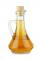How 0.5 liters of 8% vinegar diluted to a concentration of 20 hundredths % of vinegar? How many liters of water must be pour?
2. PineHow much of a mixed forest rangers want to cut down, if their head said: "We only cut down pine trees, which in our mixed forest 98%. After cut pine trees constitute 94% of all trees left."
3. Probability - tickets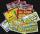What is the probability that when you have 25 tickets in 5000 that you wins the first (one) prize?
4. PersonsPersons surveyed:100 with result: Volleyball=15% Baseball=9% Sepak Takraw=8% Pingpong=8% Basketball=60% Find the average how many like Basketball and Volleyball. Please show your solution.
5. PharmacyAt the pharmacy are in one container 20% solution in the second 50% solution of disinfectant. They need to prepare 4 L of 48-percent solution. What amount of solution from each container is needed to mix?
6. Vinegar mixing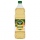We mix 70g water and 50g 8% vinegar. How many percents of the solution of vinegar we produce?
7. Sum 10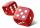What is the probability that two dice throw twice in a row will result the sum of 10?
8. PercentsHow many percents is 900 greater than the number 750?
9. Frameworks is badCalculate how many percent will increase the length of an HTML document, if any ASCII character unnecessarily encoded as hexadecimal HTML entity composed of six characters (ampersand, grid #, x, two hex digits and the semicolon). Ie. space as: &#x20;
10. The ballThe ball was discounted by 10 percent and then again by 30 percent. How many percent of the original price is now?
11. Profit gainIf 5% more is gained by selling an article for Rs. 350 than by selling it for Rs. 340, the cost of the article is:
12. Highway repairThe highway repair was planned for 15 days. However, it was reduced by 30%. How many days did the repair of the highway last?
13. Percentages52 is what percent of 93?
14. Theorem proveWe want to prove the sentense: If the natural number n is divisible by six, then n is divisible by three. From what assumption we started?
15. PharmacyThe pharmacy add to 3 liters of 95-percent alcohol 5 liters of 38.04 percent alcohol. How many percent pharmacy got?
16. Alcohol mixtureThree liters of 96 percent alcohol we set up a certain amount of distilled water to form a 54-percent alcohol. How many liters of distilled water were used?
17. Fruit tea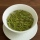Tea contains 7% of fruit components and 12% of sugar in this component. How many percents of sugar is represented in the whole tea?